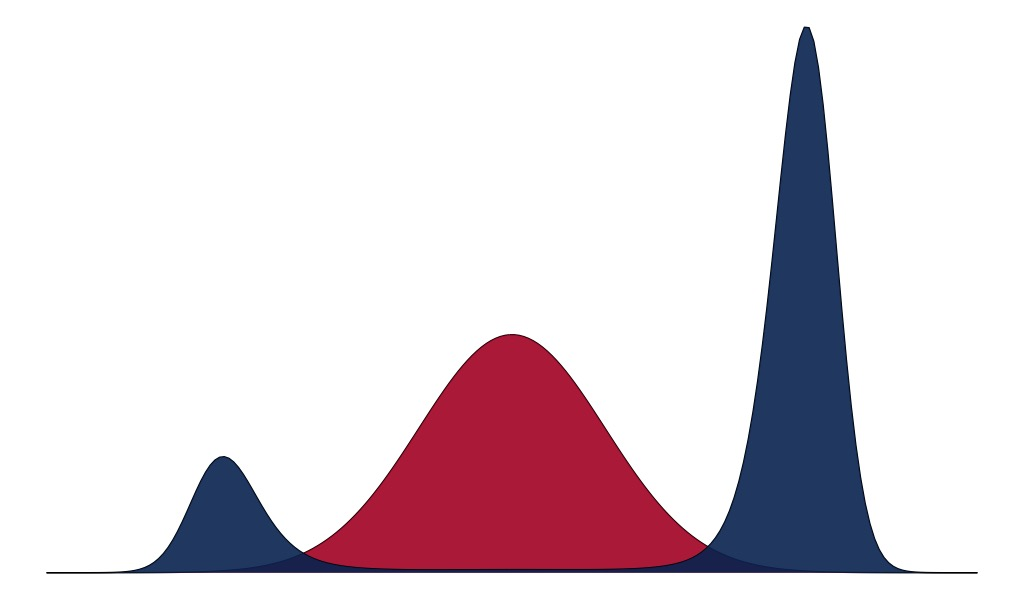# Minimum relative entropy and Generalized BayesDefine the relative entropy of a probability distribution $$\nu$$ with respect to reference measure $$\rho$$ or Kullback-Leibler divergence of $$\nu$$ to $$\rho$$ as

$D_{\text{KL}}(\nu || \rho) = - \mathrm{E}_\nu \left[ \log \frac{d\rho}{d \nu} (\theta) \right]$

The usual Bayesian update can be seen as an unconstrained minimization problem

\begin{aligned} & \underset{\nu}{\text{minimize}} && D_{\text{KL}}\left(\nu || P \right) \end{aligned}

where $$P$$ is the posterior measure induced by a prior density $$d\pi(\theta)$$ and a sampling density $$p(y | \theta)$$.

$dP(\theta) = \frac{p(y | \theta)d\pi(\theta)}{Z}$

With some re-arrangement, it can be shown that an equivalent formulation of the problem is

\begin{aligned} & \underset{\nu}{\text{minimize}} && \mathrm{E}_\nu \left[ \ell(\theta, y) \right] + D_{\text{KL}}\left(\nu || \pi \right) \end{aligned}

where $$\ell(y, \theta) = -\log p(y | \theta)$$. One interpretation of this update is that we are minimizing the expected “loss” over $$\nu$$, plus a “regularization” term that pulls us back to the prior.

Of course, since

$D_\text{KL}\left( \nu || P \right) = 0 \iff \nu = P$

the solution to the variational problem is $$P$$ itself when the class of distributions over which one optimizes is unrestricted. Most methods under the umbrella of “Variational Inference” instead tackle this minimization problem when $$\nu$$ is assumed to be from a parametric family, which may not be the parametric family to which the true posterior belongs.

## Generalized Bayes

Generalized Bayes procedures augment the loss function in the Bayesian update outlined above. In other words, they replace the negative log sampling density with a more general loss function $$L(\theta, y)$$ to produce problems of the form

\begin{aligned} & \underset{\nu}{\text{minimize}} && \mathrm{E}_\nu \left[ L(\theta, y) \right] + D_{\text{KL}}\left(\nu || \pi \right) \end{aligned}

The loss function $$L$$ can be designed to encourage certain desired behaviors of the posterior density. For instance, one can write

$L(\theta, y) = \ell(\theta, y) + \sum_{k=1}^K \lambda_k F_k(\theta)$

and then tune the weightings $$\boldsymbol{\lambda}$$ to match the desired relative encouragement of the behaviors implied by each $$F_k$$. If $$F_k$$ is a positive function everywhere in $$\theta$$, then the optimal measure $$\nu_\boldsymbol{\lambda}^\ast$$ will be such that

$\mathrm{E}_{\nu_\boldsymbol{\lambda}^\ast} \left[ F_k (\theta) \right] \rightarrow 0~~\text{as}~~\lambda_k \rightarrow \infty$

However, if $$F_k$$ is not positive everywhere in $$\theta$$, then no such relationship exists.

## Variational Bayes with equality constraints

The objective function that defines the Generalized Bayes procedure can also be motivated from the point of view of constrained optimization on the space of probability distributions.

Consider the problem of finding a measure $$\nu$$ that minimizes the relative entropy to the posterior $$P$$ subject to equality constraints on the $$\nu$$-expectation of certain functions $$\mathbf{F}(\theta) = (F_1(\theta), F_2(\theta), \dots, F_K(\theta))$$. The problem statement is

\begin{aligned} & \underset{\nu}{\text{minimize}} && D_{\text{KL}}\left(\nu || P \right) \\ &\text{subject to} && \mathrm{E}_{\nu} \left[ F_k(\theta) \right] = 0, \; k = 1, \dots, K.\\ & && \int d \nu(\theta) = 1 \end{aligned}

The objective is convex in the measure $$\nu$$, and the equality constraints are affine in $$\nu$$. Hence, the above is a convex program with an infinite number of variables and a finite number of constraints. The Lagrangian function of this problem is

$\mathcal{L}(\nu, \boldsymbol{\lambda}, \eta) = D_\text{KL}(\nu, \rho) + \sum_{k=1}^K \lambda_k \left[ \int F_k(\theta) d\nu(\theta) \right] + \eta \left[ \int d\nu(\theta) - 1 \right]$

where $$\boldsymbol{\lambda}, \eta$$ are the Lagrange multipliers or dual weights.

The minimizer of the objective belongs to a family of solutions to $$\nabla_{\nu} \mathcal{L} = 0$$, which are indexed by the dual weights $$\boldsymbol{\lambda}, \eta$$. To characterize this family, it is convenient to pass to the Donsker-Varadhan variational representation of relative entropy:

$D_{\text{KL}}(\nu || P) = \underset{g}{\sup}~ \int g(\theta) d\nu(\theta) - \log \int e^{g(\theta)} dP(\theta)$

which implies that the derivative of $$D_{\text{KL}}(\nu || \rho)$$ with respect to the measure $$\nu$$ is $$g^*$$, the function $$g$$ that attains the supremum in the representation above. Similarly, the derivative of $$\int F_k(\theta) d\nu(\theta)$$ with respect to $$\nu$$ is $$F_k(\theta)$$. Hence, $$\nabla_{\nu} \mathcal{L} = 0$$ implies

$g^*(\theta) = -\sum_{k=1}^K \lambda_k F_k(\theta) - \eta$

The theory of convex duality tells us that the minimum of the objective is reached at

$\underset{\boldsymbol{\lambda}, \eta}{\sup}~ \underset{\nu}{\inf} ~\mathcal{L}(\nu, \boldsymbol{\lambda}, \eta)$

Re-writing the Lagrangian in terms of $$g^*$$ we see that maximizing over $$\boldsymbol{\lambda}, \eta$$ is equivalent to minimizing

$\phi(\boldsymbol{\lambda}, \eta) = \int e^{-\sum_{k=1}^K \lambda_k F_k(\theta) } dP(\theta)$

where $$\eta$$ is determined by the optimal value $$\boldsymbol{\lambda}^*$$.

In the variational representation of relative entropy, the maximizing function is

$g^* (\theta) = \log \frac{d \nu}{d P} (\theta)$

Hence, the optimal measure $$\nu^*$$ has the form

$\frac{d \nu^*}{d P}(\theta) = e^{-\boldsymbol{\lambda}^{*\top} \mathbf{F}(\theta) - \eta(\boldsymbol{\lambda}^*)}$

Moving back to the Lagrangian—and ignoring the constraint associated with the dual variable $$\eta$$—we see that

\begin{aligned} \mathcal{L}(\nu, \boldsymbol{\lambda}) &= D_\text{KL}(\nu || P) + \sum_{k=1}^K \lambda_k \left[ \int F_k(\theta) d\nu(\theta) \right] \\ &= D_\text{KL}(\nu || P) + \mathrm{E}_\nu \left[ \sum_{k=1}^K \lambda_k \left( F_k(\theta) \right) \right] \\ &= \mathrm{E}_\nu \left[ \sum_{k=1}^K \lambda_k \left( F_k(\theta) \right) \right] + \mathrm{E}_\nu \left[ \ell(\theta, y) \right] + D_\text{KL}(\nu || \pi) \\ &= \mathrm{E}_\nu \left[ \tilde{L}(\theta, y)\right] + D_\text{KL}(\nu || \pi) \end{aligned}

where

$\tilde{L}(\theta, y) = \ell(\theta, y) + \sum_{k=1}^K \lambda_k F_k(\theta)$

which is a weighted loss function of the type considered in the Generalized Bayes update.

From here it is clear that the family of Generalized Bayes updates indexed by $$\boldsymbol{\lambda}$$ is a family of “suboptimal” solutions to the dual problem produced by the constrained variational Bayesian update. Depending on the value of $$\boldsymbol{\lambda}$$, the equality constraints implied by each $$F_k$$ will enforced to a greater or lesser extent.